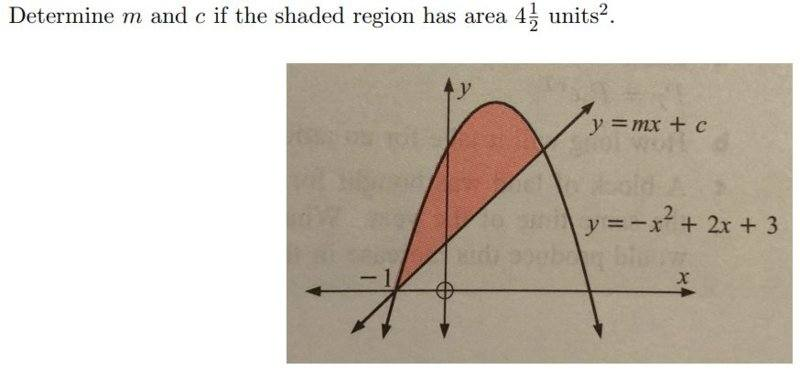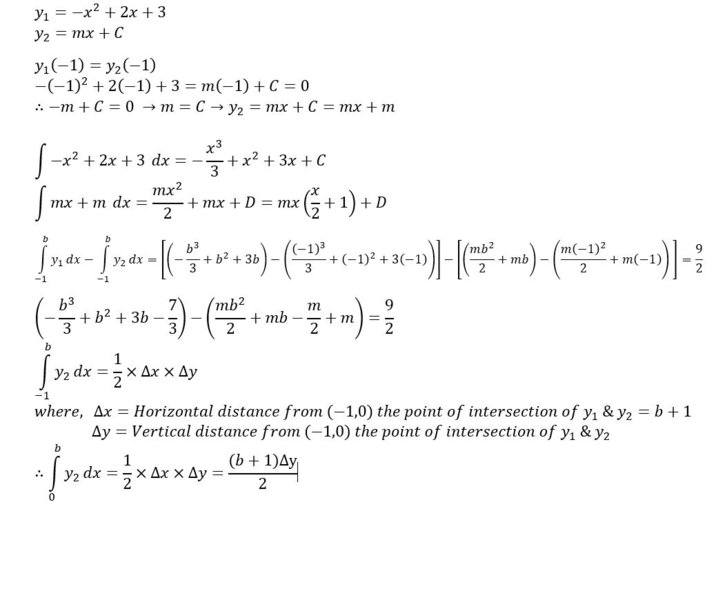# Determine m and c if the shaded region has area 4.5 units^2

## Homework Statement## The Attempt at a SolutionThe above is my attempt after playing around with the question for about an hour. I don't seem to know how to eliminate either Δy or b in order to solve for m. Any help is much appreciated. Thanks in advance.

#### Attachments

Homework Helper
Gold Member
It would help to calculate the point, (x coordinate) where the line ## y=mx+m ## intersects ## y=-x^2+2x+3 ##. Then the integral for the area would be the difference of two definite integrals. One place is (-1,0), but you need to find the other location (in terms of ## m ##).

•gex
fresh_42
Mentor
2021 Award
You have ##y=mx+m## for the straight. Why don't you calculate the right intersection point and work with definite integrals?

•Homework Helper
Gold Member
Thank you for your responses fresh_42 and Charles. I did what you suggested and came up with this.View attachment 231992
I'm unsure as to how to use this information to make my integrals definite.
So ## x=-m+3 ##. You integrate from ## x=-1 ## to ## x=-m+3 ##. That should be obvious.

•gex
Homework Helper
Gold Member
Additional comment: Once you use the limits on the definite integral, the rest is really an exercise in algebra. The result is a 3rd order equation in ## m ## that has one simple root, and two other (complex) roots that are extraneous.

Last edited:
•gex
the rest is really an exercise in algebra
That is what I'm finding very difficult right now. I've been trying to solve for m myself but can't seem to do it. I used an online calculator and the provided root isn't an integer which leads me to believe I've gone wrong somewhere else in my algebra.

Homework Helper
Gold Member
That is what I'm finding very difficult right now. I've been trying to solve for m myself but can't seem to do it. I used an online calculator and the provided root isn't an integer which leads me to believe I've gone wrong somewhere else in my algebra.
The algebra is not the easiest. Here's what I got after multiplying both sides by ## -6 ## (originally it was ## -\frac{m^3}{6 }+... ##) : ## \\ ## ## m^3-12m^2+48m-37=0 ##. ## \\ ## See if you can work to that result. ## \\ ## This equation readily factors with one simple root. My guess is that you got something slightly different.

•gex
Here's what I got after multiplying both sides by −6

Your equation is much cleaner than what I got and yields a root of m=1. Thank you so much for your help Charles, I will keep playing with the algebra in mine until it resembles your equation.

••